Assessment Questions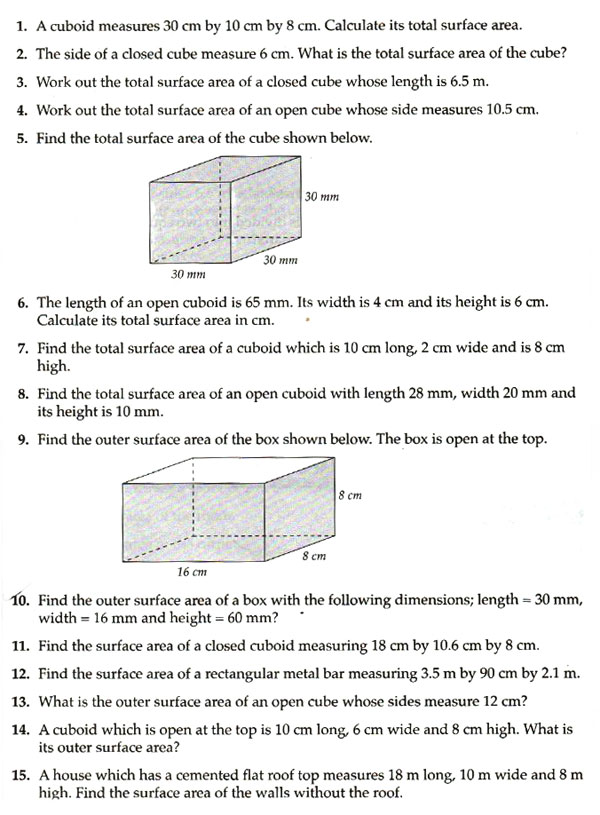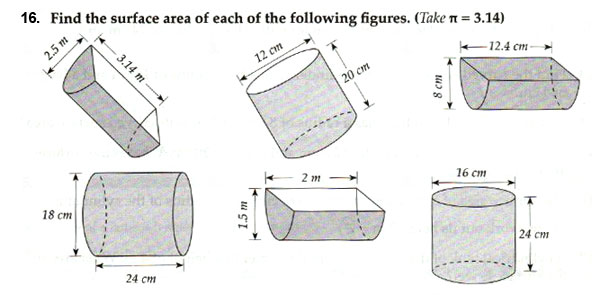17. Calculate the surface Area of the following closed cylinder.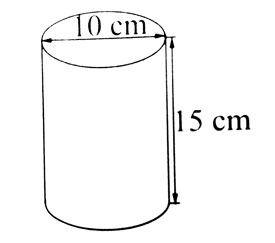18. Calculate the surface Area of the following closed cuboids.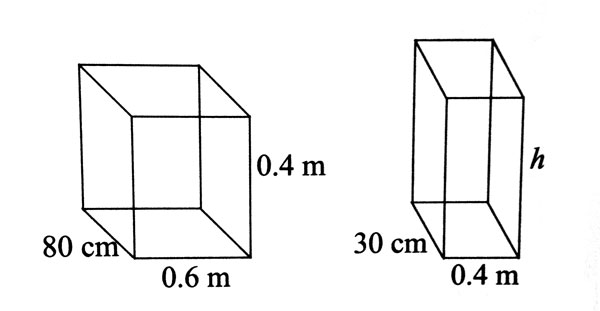19. Find the surface area of the following figures.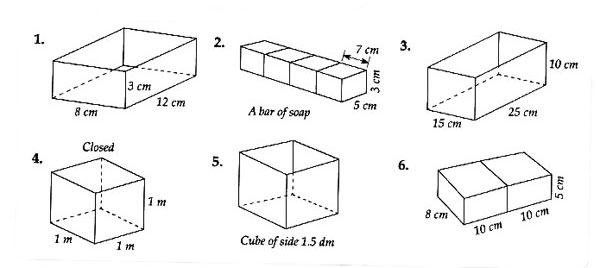20. Find the outer surface area of a box with the following dimensions; Length 30 mm, width 16 mm and height 60 mm.

21. Find the surface area of each of the following figures. (Take pi as 3.14)

22. A metal rod 60 cm long has a diameter of 1.4 cm. Find its surface area.

23. A cardboard map holder is in the shape of a cylinder open at one end. If it has a radius of 8.4 cm and a height of 60 cm. What is its outer surface area?

24. A petrol drum is 2.5 m long and has a diameter of 60 cm. If a drum is rolled in a straight line until it completes 100 revolutions, What area will it cover? Pi = 3.14.

25. A 4 m long cylinder has a volume of 98.56 m3 Calculate its surface area if it is closed on both ends.

26. The surface area of a closed cylinder is 2112 cm2. If the radius of the cylinder is 14 cm, work out its height. $$(\pi = {22\over7})$$